Variable Voltage Regulators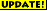29 Jan 05 - Heatsink requirements moved to a separate page.

This design is intended to produce a variable, regulated, voltage between 1.2V and 37V. The LM 317T regulator is guaranteed for a minimum output current of 1.5A. The design is quite simple, the only complication being the working out of the resistor values for RB.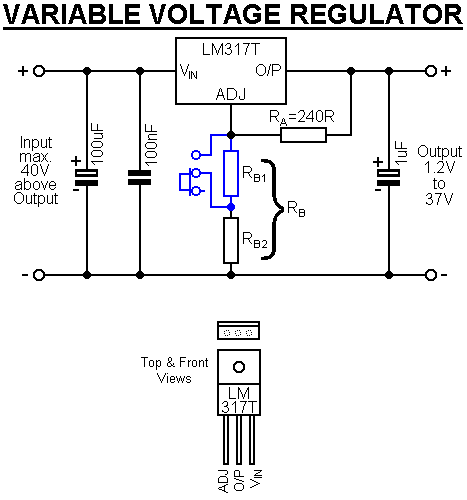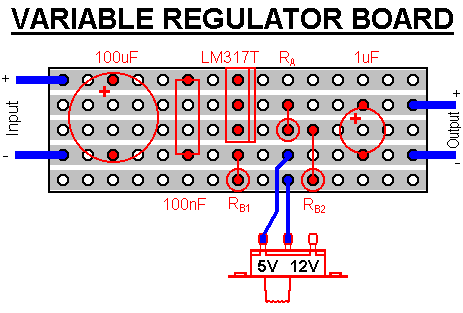This design shows a switch that is used to alternate between 2 resistances, giving 2 output voltages. If this facility is not required, RB1 and the switch can be removed. The lower leg of RB2 (shown as the body above) would also need to be moved up onto the negative output rail. Alternatively, the switch and RB1 can be replaced by a suitable variable resistor to give adjustment of the voltage.

#### Output Voltage Calculation

The output voltage is determined by the equation: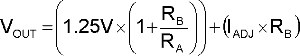The error in omitting the IADJ x RB value is usually quite small (around 0.13V at a 12V output, and only 0.34V at 37V output) and it can be therefore be ignored in most applications, which leaves: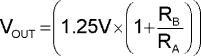orIn the design above shows an RA value of 240R, which is the standard value, although other values can be used.

If accuracy is really important the value of IADJ needs to taken into account and is typically 50µA (maximum 100µA), which can be derived into an alternate equation:As an example I'll consider a regulator built to provide a voltage output switchable between 5V and 12V and to provide 1.5A. It will operate in a well ventilated case, which reaches a maximum internal temperature (TAMB) of 25°C. The input voltage would need to be above the 12V maximum and a therefore a 15V transformer is selected.

For simplicity, and at this low voltage the error is small, I'll use the simplified equation above. For the 5V output RB = 720R and for the 12V output RB = 2064R

The 720R resistance can be made by putting 680R and 39R resistors in series. The increase is resistance required is 2064R - 720R = 1344R, which can be achieved by putting 1000R (1k) and 330R resistors in series or 2 x 2.7k resistors in parallel (1.35k).

#### Temperature Considerations

Another thing that needs to be taken into account is ensuring that the regulator doesn't overheat. It is fitted with an internal over temperature cut-out, but you don't want the power dying when you trying to use the output. To determine whether you need to use a heatsink, click here